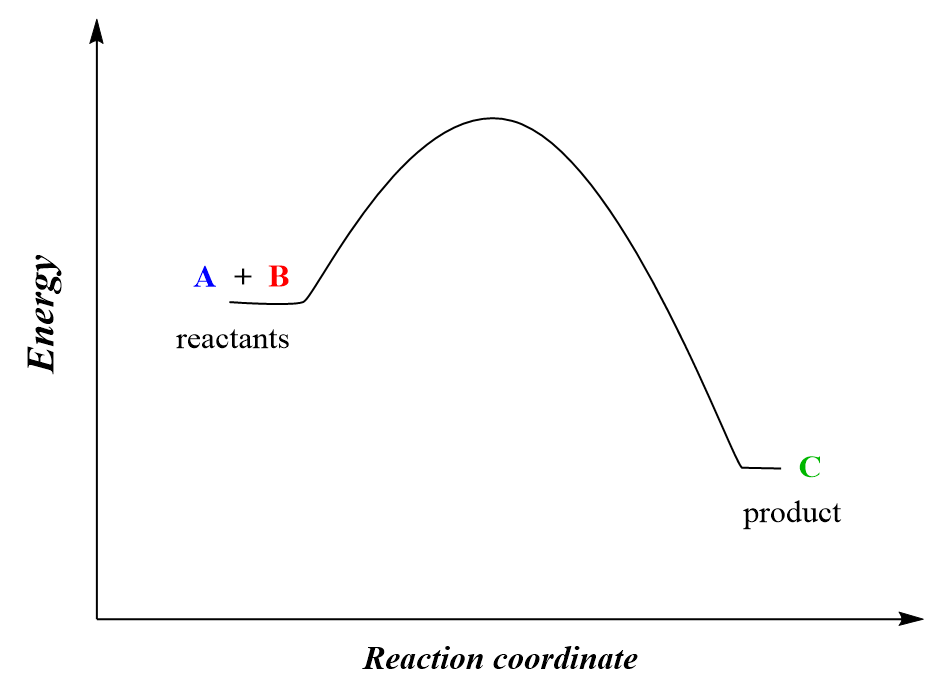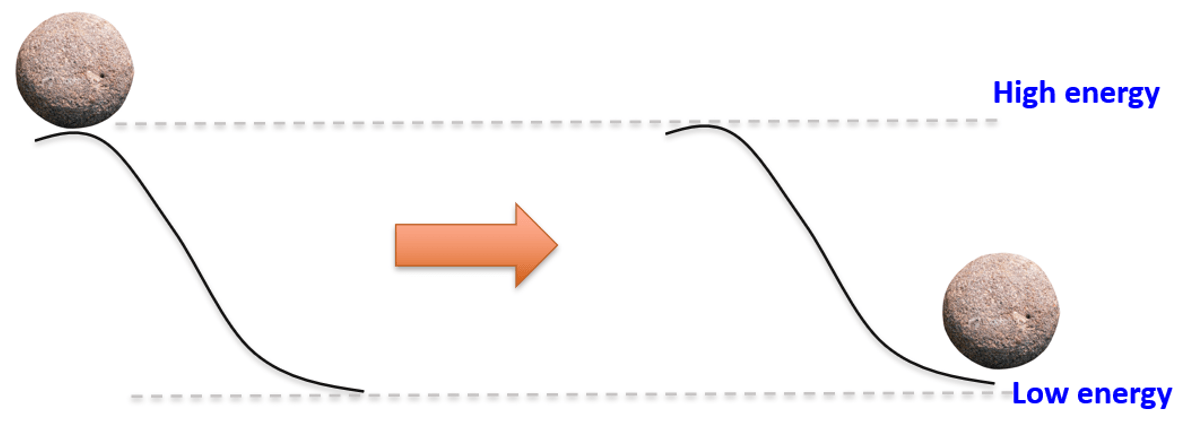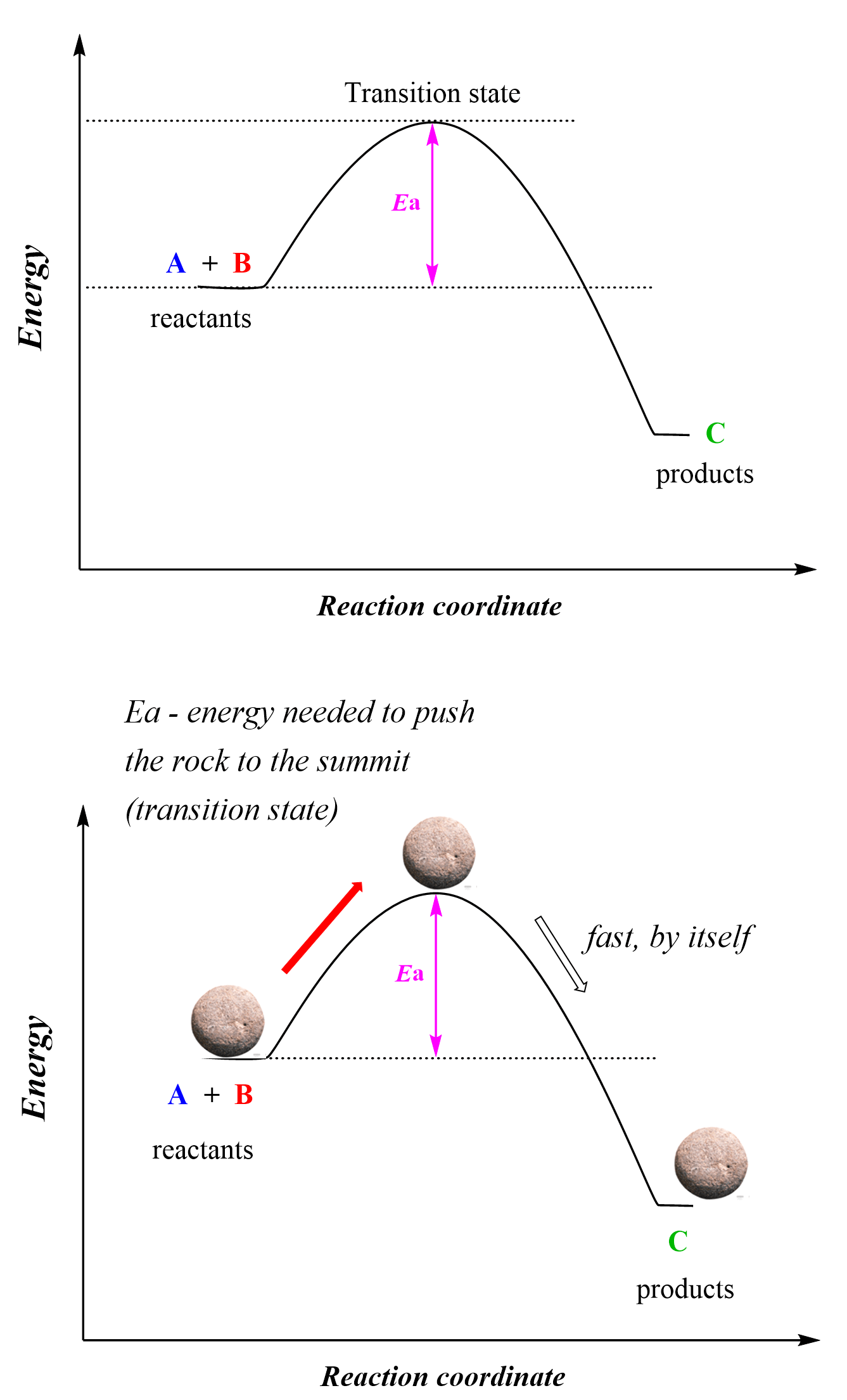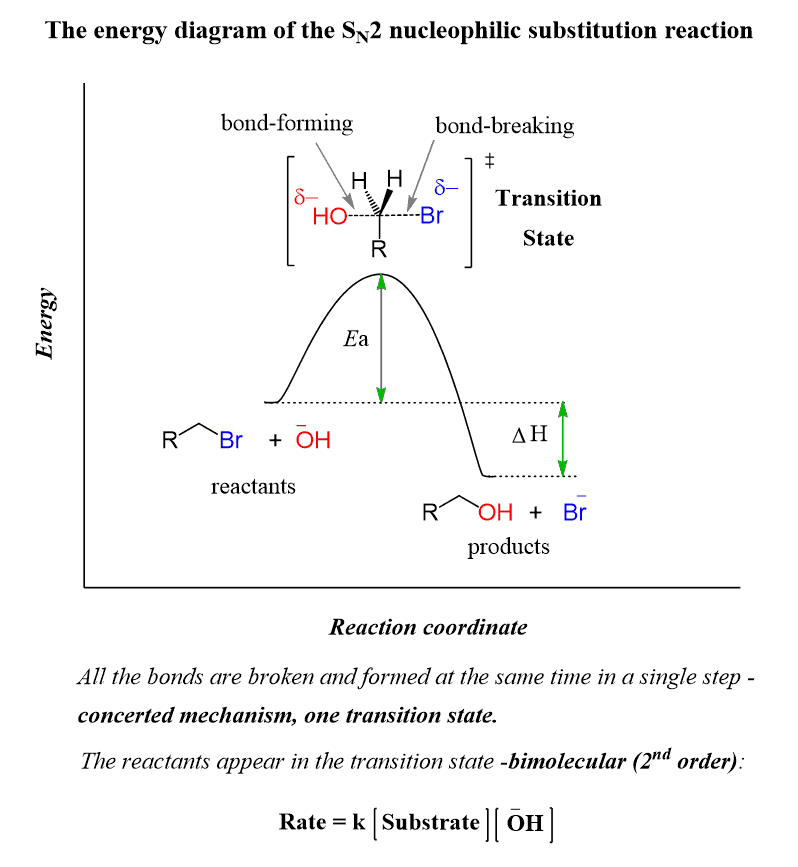## General Chemistry

A chemical reaction between two molecules occurs when they collide with proper orientation and sufficient kinetic energy. During the collision, the kinetic energy is used to stretch, bend, and ultimately break bonds, initiating the chemical reaction where new bonds and therefore, new compounds are formed.  This model was first suggested in 1888 by a Swedish chemist Svante Arrhenius who emphasized that in order for the molecules to react they must possess a certain minimum amount of energy which is called the activation energy, Ea, and is characteristic of each reaction at given conditions.

The concept of activation energy is best shown on energy diagrams which show the plot of the reaction coordinate and time. The reaction coordinate is essentially the state of the reaction that shows what components and at what energy level are present at a given time of the reaction.

For example, the reaction between molecules A and B leading to the formation of C and D can be shown using the energy diagram below:

A + B → CThe energy diagram tells us that the products have lower energy than the reactants, so one might expect that this process should occur by itself because remember, all the systems/reactions/processes tend to move in the direction with lower energy. Dropping any object, turning the sand watch, an avalanche are a few examples of the system going to a lower potential energy state.However, the reactants need to have sufficient energy to pass the hump and become products which is the activation energy:So, activation energy is the difference between the energy of the reactants and the energy of the transition state. The activation energy defines how fast or slow a reaction occurs and this is what we call the kinetics of the reaction. The highest energy point of the reaction is achieved when the reactive species overcome the activation energy barrier and this is the transition state of the reaction where some bonds are partially broken and new ones are partially formed.

For example, consider the following reaction (SN2 reaction you will cover in organic chemistry) between an alkyl halide and a hydroxide. The highest energy point is the transition state where the C-Br bond is broken and a new, C-O bond is being formed. To form a transition state (activated complex), the molecules had enough kinetic energy, collided, and overcame the activation energy (Ea) barrier.So, remember, just because the products have lower energy, meaning they are more stable (the thermodynamics of the reaction) does not mean the reaction is going to occur right away. Yes, the reaction will have the tendency to occur, however, how fast (kinetics) it occurs will depend on the activation energy. The classical example is the combustion of flammables such as gasoline. It is an extremely favorable reaction in terms of thermodynamics because there is a lot of energy released which means the products are more stable than the reactants. However, luckily gas tanks do not explode by themselves because the molecules need activation energy which can, for example, be a spark.

# Activation Energy and Reaction Rate

There are two main approaches to increasing the rate of a chemical reaction. One is lowering the activation energy which means less energy will be required from molecules to overcome the activation energy barrier. And the second is increasing the temperature which increases the kinetic energy of the molecules and thus, they can pass the activation energy barrier.

Lowering the activation energy is achieved by using a catalyst, while the effect of temperature is described in detail by the Arrhenius equation. These will be the topics for our next discussions.

Here is a 77-question, Multiple-Choice Quiz on Chemical Kinetics:

Check Also

### 2 thoughts on “Activation Energy”

1.•